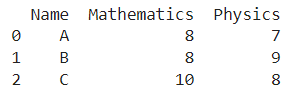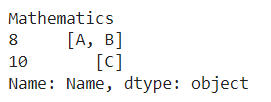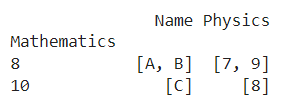# How To Group Dataframe Rows Into List In Pandas Groupby

A DataFrame is the primary data structure of the Pandas library in Python and is commonly used for storing and working with tabular data.

A common operation that could be performed on such data is to group dataframe rows into a list in the DataFrame to work with the information in a better manner using groupby.

To start working with Pandas, we first need to import it :

``import pandas as pd``

## Running Example

Let us understand this operation with the help of an example. Consider the following DataFrame containing 3 students with names A, B, and C and their corresponding marks (out of 10) for two subjects, Mathematics and Physics.Code snippet for generating the above DataFrame :

### Python 3 Code :

``````# Importing required libraries
import pandas as pd

# Dictionary for our data
data = {'Name' : ['A', 'B', 'C'], 'Mathematics' : [8, 8, 10], 'Physics' : [7, 9, 8]}

# DataFrame for the dictionary
df = pd.DataFrame(data=data)

# PrintingÂ
print(df)``````

Here, data is a dictionary that we created to initialize the DataFrame. For this, we use the DataFrame() function of the Pandas library which takes the dictionary as an argument and returns the required DataFrame.

Now, let’s say we need to group the rows into a list using pandas groupby. Say the column using which we need to group by is the mathematics column. The resulting output would look like this:Let us look at different ways of performing this operation on a given DataFrame :

## 1. Group Dataframe Rows Using the groupby() and apply() function

In this method, we use the groupby() function along with the apply() function to get a list of rows after grouping them using the desired column, here mathematics.

Inside the groupby function, we pass the column of interest, mathematics, as a parameter. Next, we use square brackets along with the column label of the name passed to access the name column. After this, we use the apply() function with the parameter as a list to convert the result to lists.

The changes are not made in an in place manner therefore reassignment is required. Let us take a look at the corresponding code snippet and generated output for this method:

``````# Importing required libraries
import pandas as pd

# Dictionary for our data
data = {'Name' : ['A', 'B', 'C'], 'Mathematics' : [8, 8, 10], 'Physics' : [7, 9, 8]}

# DataFrame for the dictionary
df = pd.DataFrame(data=data)

# Performing the operation
df = df.groupby('Mathematics')['Name'].apply(list)

# PrintingÂ
print(df)``````

Output :Instead of using [‘Name’] to access the name column, we could also simply use the dot operator to get the same result. Let us take a look at the corresponding code snippet and generated output for this method:

``````# Importing required libraries
import pandas as pd

# Dictionary for our data
data = {'Name' : ['A', 'B', 'C'], 'Mathematics' : [8, 8, 10], 'Physics' : [7, 9, 8]}

# DataFrame for the dictionary
df = pd.DataFrame(data=data)

# Performing the operation
df = df.groupby('Mathematics').Name.apply(list)

# PrintingÂ
print(df)``````

Output :## 2. Group Dataframe Rows Using the .agg() function

In this method, we use the .agg() function to combine the contents of getting a list of rows after grouping them using the desired column, here mathematics. We first use the dataframe.groupby() function as described in the previous method along with the parameter as the label of the desired column, mathematics.

Then, we apply the agg() function to this with the parameter as a list to get the desired output. The changes are not made in an in place manner therefore reassignment is required. Let us take a look at the corresponding code snippet and generated output for this method:

``````# Importing required libraries
import pandas as pd

# Dictionary for our data
data = {'Name' : ['A', 'B', 'C'], 'Mathematics' : [8, 8, 10], 'Physics' : [7, 9, 8]}

# DataFrame for the dictionary
df = pd.DataFrame(data=data)

# Performing the operation
df = df.groupby('Mathematics').agg(list)

# PrintingÂ
print(df)``````

Output :## Conclusion

In this topic, we have learned to group the rows of an existing Pandas DataFrame into lists using pandas groupby, following a running example of test scores of students in different subjects, thus giving us an intuition of how this concept could be applied in real-world situations. Feel free to reach out to info.javaexercise@gmail.com in case of any suggestions.

Trending